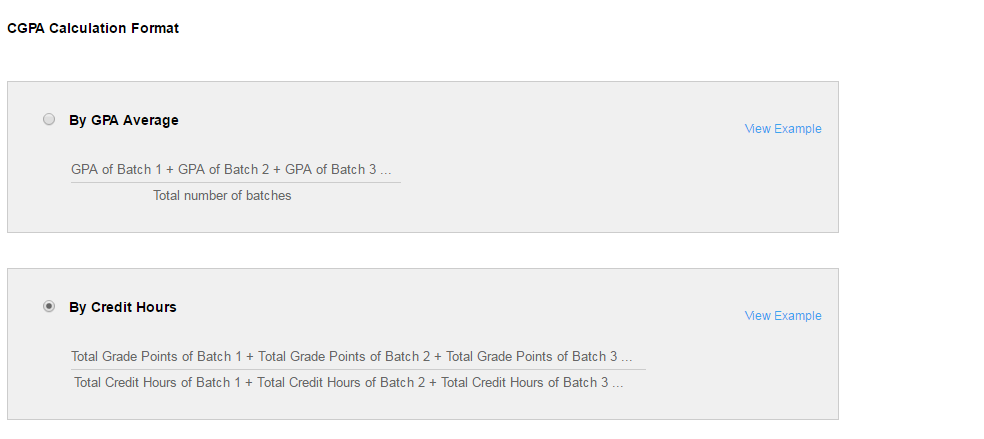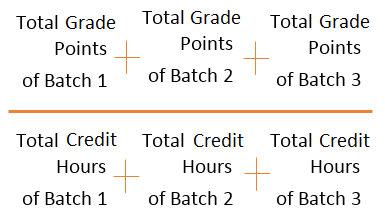Fedena offers the following two methods to calculate CGPA:

• By GPA Average

• By Credit Hours### By GPA Average

The formula used is:### By Credit Hours

The formula used is:Example

Let’s assume that credit points are assigned as follows.

 A = 4 A- = 3.7 B+ = 3.3 B = 3 B- = 2.7 C+ = 2.3 C = 2 C- = 1.7 D+ = 1.3 D = 1 D- = .7 F = 0

Calculation of Shaun’s GPA for Batch 1 will be as follows.

 Subject (Batch Subjects) Credit Hours (Assigned to each subject) Grade (Secured by student for subject) Grade Points (Credit points × Credit hours) English 3 A 3 × 4 = 12 Arabic 1 B 1 × 3 = 3 Mathematics 3 C 3 × 2 = 6 Social 3 F 3 × 0 = 0

Total Credit Hours = 3 + 1 + 3 + 3 = 10

Total Grade Points = 12 + 3 + 6 + 0 = 21

Shaun’s GPA for Batch 1 = 21/10 = 2.10

Calculation of Shaun’s GPA for Batch 2 will be as follows.

 Subject (Batch Subjects) Credit Hours (Assigned to each subject) Grade (Secured by student for subject) Grade Points (Credit points × Credit hours) English 3 A 3 × 4 = 12 Arabic 1 B 1 × 3 = 3 Mathematics 3 B 3 × 3 = 9 Social 3 A 3 × 4 = 12

Total Credit Hours = 3 + 1 + 3 + 3 = 10

Total Grade Points = 12 + 3 + 9 + 12 = 36

Shaun’s GPA for Batch 2 = 36/10 = 3.60

Calculating Shaun’s CGPA by GPA Average:= (2.10 + 3.60)/2 = 2.85

Calculating Shaun’s CGPA by Credit Hours:Where:

• Grade Point is Credit Hours assigned to the Subject × Credit Points corresponding to the grade secured by the student

• Total Grade Point is the Sum of (Grade Points of all subjects of batches under a course)

• Total Credit Hours is the Sum of (Credit hours of all subjects of batches under a course)

Shaun’s CGPA = (21 + 36) / (10 + 10) = 2.85